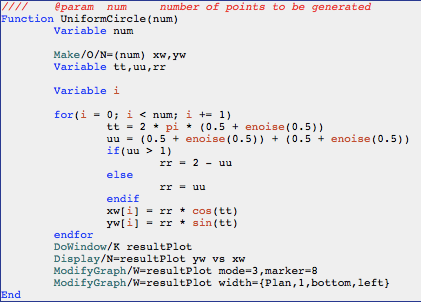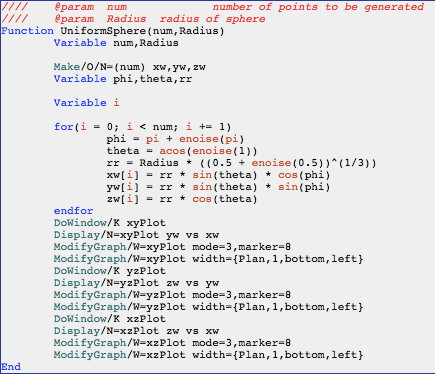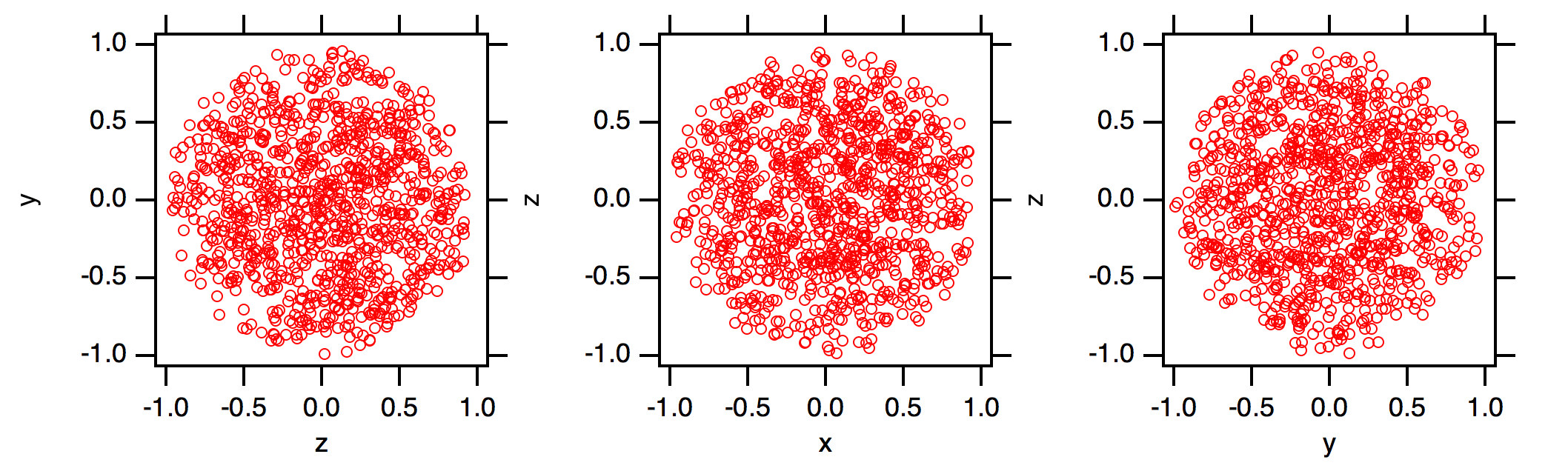I needed to generate a uniform random distribution of points inside a circle and, later, a sphere. This is part of a bigger project, but the code to do this is kind of interesting. There were no solutions available for IgorPro, but stackexchange had plenty of examples in python and mathematica. There are many ways to do this. The most popular seems to be to generate a uniform random set of points in a square or cube and then discard those that are greater than the radius away from the origin. I didn’t like this idea, because I needed to extend it to spheroids eventually, and as I saw it the computation time saved was minimal.

Here is the version for points in a circle (radius = 1, centred on the origin).This gives a nice set of points, 1000 shown here.And here is the version inside a sphere. This code has variable radius for the sphere.The three waves (xw,yw,zw) can be concatenated and displayed in a Gizmo. The code just plots out the three views.My code uses var + enoise(var) to get a random variable from 0,var. This is because enoise goes from -var to +var. There is an interesting discussion about whether this is a truly flat PDF here.

This is part of a bigger project where I’ve had invaluable help from Tom Honnor from Statistics.

This post is part of a series on esoterica in computer programming.